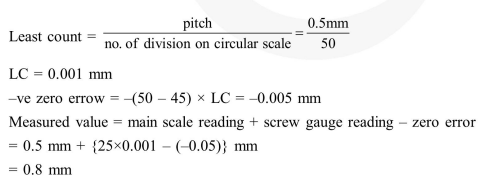# A screw gauge with a pitch of`
Question:

A screw gauge with a pitch of $0.5 \mathrm{~mm}$ and a circular scale with 50 divisions is used to measure the thickness of a thin sheet of Aluminium. Before starting the measurement, it is found that when the two jaws of the screw gauge are brought in contact, the $45^{\text {th }}$ division coincides with the main scale line and that the zero of the main scale is barely visible. What is the thickness of the sheet if the main scale reading is $0.5 \mathrm{~mm}$ and the $25^{\text {th }}$ division coincides with the main scale line ?

1. $0.50 \mathrm{~mm}$

2. $0.75 \mathrm{~mm}$

3. $0.80 \mathrm{~mm}$

4. $0.70 \mathrm{~mm}$

Correct Option: , 3

Solution: